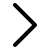BlogArticles

# Weighted Average Cost of Capital (WACC) or discount rate analysisUnderstanding the inner workings of financial concepts like the Weighted Average Cost of Capital (WACC) and discount rates can give you a critical advantage when making business decisions. This article aims to break down the concepts, calculations, and utility of these significant financial tools.

## What is WACC and how is it used?

The Weighted Average Cost of Capital, commonly known as WACC, represents the average rate a company is expected to pay to finance its assets, either through debt or equity. It serves as the minimum return that a company must earn on its existing asset base to satisfy its creditors, owners, and other providers of capital.

The required return for a particular investor is composed of two parts:

1) the risk-free return, which is typically the interest rate on a AAA-rated government bond with a similar maturity, and

2) a risk premium, which compensates investors for taking on additional risk. The higher the risk premium, the higher the required return.

## Why is WACC used as a Discount Rate?

WACC is often used as a discount rate because it encapsulates the risk associated with a specific company's operations. The WACC indicates the expected cost of new capital, which aligns with future cash flows—a primary factor that should match with the discount rate in a discounted cash flow (DCF) analysis. The WACC offers a comprehensive measure of the risk and the cost of capital, making it a suitable discount rate.

## What Weightings Should I Use in WACC?

In WACC, the weightings refer to the proportion of each financing category (debt and equity) in a company's capital structure. These weights are calculated using the market values of equity and debt, thus reflecting the company's targeted or actual capital structure.

## How are the Different Components of WACC Calculated?

The WACC calculation includes the cost of equity, the cost of debt, and the respective proportions of each in the company's capital structure.

The cost of equity can be estimated using models like the Capital Asset Pricing Model (CAPM), which incorporates the risk-free rate, beta (a measure of stock volatility), and expected market return.

The cost of debt is typically the interest rate the company would pay if it issues new debt, and it is adjusted for the tax shield (since interest expenses are tax-deductible).

The formula for WACC is:

WACC = (E/V * Re) + (D/V * Rd * (1 - Tc))

Where:
E = market value of equity
V = total market value of equity and debt
Re = cost of equity
D = market value of debt
Rd = cost of debt
Tc = corporate tax rate

## How is WACC used in Connection with the Discounted Cash Flow (DCF) Model and Other Projections?

In the DCF model, future cash flows are discounted back to their present value using a discount rate, often the WACC, to ascertain an investment's attractiveness. When the present value of these cash flows is higher than the investment cost, the investment may be seen as worthwhile. Thus, the WACC serves as the bridge between future value and present value in various projections.

## What is the Difference Between WACC and Discount Rate?

While WACC can be used as a discount rate, the two are not always the same. The discount rate reflects the risk associated with future cash flows, while the WACC represents the average cost of a company's capital. A company might use different discount rates for different projects, reflecting their unique risk profiles, but the WACC remains constant across the company as it reflects its overall risk and financing cost.

## How can I streamline my WACC analysis?

In the financial landscape, understanding and correctly applying WACC and discount rates can seem challenging. That's where Comparables.ai can help. With the Comparables.ai platform, you can effortlessly calculate the unlevered beta for a public company peer group, a crucial input in calculating the WACC. Calculating WACC has never been easier or more accurate.